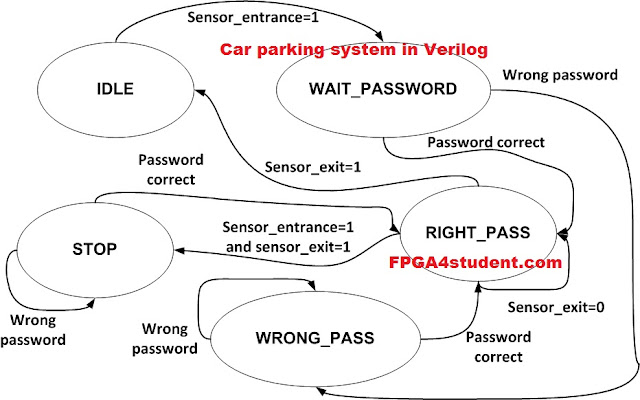# Verilog code for Car Parking System

## This simple project is to implement a car parking system in Verilog. The Verilog code for the car parking system is fully presented.

#### In the entrance of the parking system, there is a sensor which is activated to detect a vehicle coming. Once the sensor is triggered, a password is requested to open the gate. If the entered password is correct, the gate would open to let the vehicle get in. Otherwise, the gate is still locked. If the current car is getting in the car park being detected by the exit sensor and another the car comes, the door will be locked and requires the coming car to enter passwords.### Verilog code for the car parking system:

```// fpga4student.com FPGA projects, Verilog projects, VHDL projects
// Verilog project: Verilog code for car parking system
`timescale 1ns / 1ps
module parking_system(
input clk,reset_n,
input sensor_entrance, sensor_exit,
output wire GREEN_LED,RED_LED,
output reg [6:0] HEX_1, HEX_2
);
parameter IDLE = 3'b000, WAIT_PASSWORD = 3'b001, WRONG_PASS = 3'b010, RIGHT_PASS = 3'b011,STOP = 3'b100;
// Moore FSM : output just depends on the current state
reg[2:0] current_state, next_state;
reg[31:0] counter_wait;
reg red_tmp,green_tmp;
// Next state
always @(posedge clk or negedge reset_n)
begin
if(~reset_n)
current_state = IDLE;
else
current_state = next_state;
end
// counter_wait
always @(posedge clk or negedge reset_n)
begin
if(~reset_n)
counter_wait <= 0;
counter_wait <= counter_wait + 1;
else
counter_wait <= 0;
end
// change state
// fpga4student.com FPGA projects, Verilog projects, VHDL projects
always @(*)
begin
case(current_state)
IDLE: begin
if(sensor_entrance == 1)
else
next_state = IDLE;
end
if(counter_wait <= 3)
else
begin
next_state = RIGHT_PASS;
else
next_state = WRONG_PASS;
end
end
WRONG_PASS: begin
next_state = RIGHT_PASS;
else
next_state = WRONG_PASS;
end
RIGHT_PASS: begin
if(sensor_entrance==1 && sensor_exit == 1)
next_state = STOP;
else if(sensor_exit == 1)
next_state = IDLE;
else
next_state = RIGHT_PASS;
end
STOP: begin
next_state = RIGHT_PASS;
else
next_state = STOP;
end
default: next_state = IDLE;
endcase
end
// LEDs and output, change the period of blinking LEDs here
always @(posedge clk) begin
case(current_state)
IDLE: begin
green_tmp = 1'b0;
red_tmp = 1'b0;
HEX_1 = 7'b1111111; // off
HEX_2 = 7'b1111111; // off
end
green_tmp = 1'b0;
red_tmp = 1'b1;
HEX_1 = 7'b000_0110; // E
HEX_2 = 7'b010_1011; // n
end
WRONG_PASS: begin
green_tmp = 1'b0;
red_tmp = ~red_tmp;
HEX_1 = 7'b000_0110; // E
HEX_2 = 7'b000_0110; // E
end
RIGHT_PASS: begin
green_tmp = ~green_tmp;
red_tmp = 1'b0;
HEX_1 = 7'b000_0010; // 6
HEX_2 = 7'b100_0000; // 0
end
STOP: begin
green_tmp = 1'b0;
red_tmp = ~red_tmp;
HEX_1 = 7'b001_0010; // 5
HEX_2 = 7'b000_1100; // P
end
endcase
end
assign RED_LED = red_tmp  ;
assign GREEN_LED = green_tmp;

endmodule
```

### Testbench Verilog code for car parking system:

````timescale 1ns / 1ps
// fpga4student.com FPGA projects, Verilog projects, VHDL projects
// Verilog project: Verilog code for car parking system
module tb_parking_system;

// Inputs
reg clk;
reg reset_n;
reg sensor_entrance;
reg sensor_exit;

// Outputs
wire GREEN_LED;
wire RED_LED;
wire [6:0] HEX_1;
wire [6:0] HEX_2;
// fpga4student.com FPGA projects, Verilog projects, VHDL projects
// Instantiate the Unit Under Test (UUT)
parking_system uut (
.clk(clk),
.reset_n(reset_n),
.sensor_entrance(sensor_entrance),
.sensor_exit(sensor_exit),
.GREEN_LED(GREEN_LED),
.RED_LED(RED_LED),
.HEX_1(HEX_1),
.HEX_2(HEX_2)
);
initial begin
clk = 0;
forever #10 clk = ~clk;
end
initial begin
// Initialize Inputs
reset_n = 0;
sensor_entrance = 0;
sensor_exit = 0;
// Wait 100 ns for global reset to finish
#100;
reset_n = 1;
#20;
sensor_entrance = 1;
#1000;
sensor_entrance = 0;
#2000;
sensor_exit =1;

// fpga4student.com FPGA projects, Verilog projects, VHDL projects
end

endmodule
```

1.what type of sensor are we using?

2.In this code, the sensor is modeled as a switch for simulation only.

3.I want to do this project by Gate level modeling.It is possible???

1.Absolutely can. However, you have to create state table and obtain the Boolean equations from K-maps. Then, based on the equations to write the structural code for the project.

4.What is the role of HEX_1 and HEX_2?

1.Display the state of the system

5.in this sensor exit=1 represents the car is leaving from the parking place?

1.Leaving the gate of the car park.

6.What are all the requirements for this project? h/w and s/w? TIA

7.what is the role of green_led & red_led?

8.Hi...

why it is showing green light 1 and also what about binary hex. And I tried to do RTL schematic.. It is showing failed.Can you please send the exact code if you don't have any problem. I'm using for my project.

9.i want to do these project in transistor level design, is it possible.

10.Can we implement it on fpga?

11.Which FPGA board ca be used for this program?

12.can i get flowchart of this system? because i not understand well by coding